﻿ Comparative Analysis of Higher Constellation in Intercarrier Interference Self-cancellation Orthogonal Frequency Division MultiplexingInternational Journal of Networks and Communications

p-ISSN: 2168-4936    e-ISSN: 2168-4944

2020;  10(2): 47-53

doi:10.5923/j.ijnc.20201002.03

Received: Oct. 28, 2020; Accepted: Nov. 13, 2020; Published: Nov. 28, 2020### Comparative Analysis of Higher Constellation in Intercarrier Interference Self-cancellation Orthogonal Frequency Division Multiplexing

Akintoye N. O.1, Adesina A. A.2, Salami S. A.2, Alabi T. I.2, Ojo S. I.2

2Department of Electronic and Electrical Engineering, Ladoke Akintola University of Technology, Ogbomoso, Oyo State, Nigeria

Correspondence to: Ojo S. I., Department of Electronic and Electrical Engineering, Ladoke Akintola University of Technology, Ogbomoso, Oyo State, Nigeria.
 Email: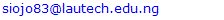Abstract

Thedemand for high data rate transmission is increasing daily and services require reliable data transmission over harsh environment. However, high data rate transmission resulting in signal overlapping at the receiver due to delay and time variation of the received signal. Orthogonal Frequency Division Multiplexing (OFDM) used to solve this problem is associated with Intercarrier Interference (ICI) that affects system performance. OFDM with ICI self-cancellation (OFDM-SC) is proposed to remove ICI generated in OFDM but suffers from poor bandwidth efficiency at low constellation size of modulation. This paper therefore, evaluate the performance of OFDM-SC with higher constellation using Bit Error Rate (BER) and Spectral Efficiency (SE). Mathematical expression for BER and SE is derived using signal strength of the received signal. The system is simulated using MATLAB R2018a to obtained the BER and SE values at different constellation size. The results obtained revealed that, OFDM-SC with 128QAM shows better performance with higher bandwidth efficiency when compared with lower constellation size such as 16QAM and 32QAM. The investigation shows that, OFDM-SC with higher constellation improve the performance of conventional OFDM.

Keywords: Orthogonal Frequency Division Multiplexing (OFDM), Inter Symbol Interference (ISI), Intercarrier Interference (ICI), Self-cancellation (SC), Spectral Efficiency (SE), Frequency Offset (OF) and Quadrature Amplitude Modulation (QAM)

Cite this paper: Akintoye N. O., Adesina A. A., Salami S. A., Alabi T. I., Ojo S. I., Comparative Analysis of Higher Constellation in Intercarrier Interference Self-cancellation Orthogonal Frequency Division Multiplexing, International Journal of Networks and Communications, Vol. 10 No. 2, 2020, pp. 47-53. doi: 10.5923/j.ijnc.20201002.03.

### 2. Related Works

In , carrier frequency offset estimation for OFDM systems using frequency domain equalization technique to combat the effect of ICI in OFDM system was carried out. The ICI for each frame was estimated by inserting frequency domain pilot symbols in each frame. The equalizer co-efficient to eliminate ICI in the frequency domain was derived from the pattern of the pilot symbol, a suitable equalizer was constructed and used to multiplied the received signal to cancel ICI. The results of the paper showed that, the technique is simple in computation when compared to other techniques such as extended Kalman filter but suffers from poor performance due to increase in side lobes of sub-carrier’s signal that resulted from multiplied the received signal with equalizer. In , Bit Error Rate (BER) improvement of OFDM system using ICI self-cancellation technique was proposed to solve the problem of ICI in OFDM. In this paper, input data symbol is modulated into a group of subcarriers with predefined coefficient such that the generated ICI signals within the subcarriers cancel each other. The generated ICI from each subcarrier mutually cancelled each other. The result of this work revealed that, the technique is efficient, simple and less complex but suffer from reduction in bandwidth efficiency as the same symbol occupies two sub-carriers. Also, in , ICI cancellation schemes for BER performance improvement of OFDM system is proposed to combat the effect of ICI in OFDM system using extended kalman filter. In this paper, the authors used two stages to solve problem of ICI. In the first stage, the frequency offset for each subcarrier was estimated by using a training sequence. In the second stage, the estimated frequency offset that followed trained sequence was then mitigated by multiplying the received signal with complex conjugate of the estimated frequency offset. The results obtained revealed that, the technique has a better performance but suffers from increase in noise resulted from multiplied the conjugate of frequency offset with the received signal. Furthermore, CFO estimation and mitigation in OFDM was carried out in [17,29] to combat the effect of ICI using maximum likelihood estimation scheme. In this paper, the frequency offsets were first statistically estimated using maximum likelihood algorithm. The estimated frequency offset was then cancelled at the receiver by multiplying the received signal with a conjugate of frequency offset. The results obtained revealed that, the proposed technique suffers from poor performance due to higher values of Bit Error Rate (BER). However, previous work on ICI cancellation in OFDM technique shows that, intercarrier interference self-cancellation technique has the best performance and very easy to implement when compared with other ICI cancellation techniques. However, the technique suffers from bandwidth inefficiency. The bandwidth consumption of intercarrier interference self-cancellation technique depends on the constellation size of modulation used. This paper therefore evaluates the effect of higher constellation in ICI self-cancellation using Bit Error Rate (BER) and Spectral Efficiency (SE).

#### 2.1. Inter-Carrier Interference (ICI) Self- Cancellation

Inter-Carrier Interference (ICI) self-cancellation technique involves modulating one data symbol onto a group of adjacent subcarriers. The modulation is with predefine coefficients such that the generated ICI signals within the group cancel each other. The weighting coefficients are designed in such a way that, the ICI caused by the channel frequency errors is minimized. In this technique, one data symbol is not modulated into a sub-carrier, but at least into two consecutive sub-carriers. If the symbol ‘y’ is modulated in to the first sub-carrier then ‘-y‘is modulated into the second sub-carrier. Therefore, the ICI generated between the two sub-carriers mutually cancels each other. The technique is suitable for multipath fading channels due to estimation that fails as the channel changes randomly. ICI self-cancellation technique is simple, effective and less complex. However, the technique leads to reduction in bandwidth efficiency as the same symbol occupies two sub-carriers [18,19,27,29].

#### 2.2. Mary Quadrature Amplitude Modulation

Quadrature Amplitude Modulation (QAM) is a digital modulation technique that modifies both the phase and amplitude of the signal simultaneously. QAM offers increased in spectral efficiency. It is accomplished by varying the amplitude of two sinusoidal waveforms that are in quadrature to each other and summing them together. This allows the effective transmission of two channels at the same frequency thereby doubling the rate at which the data is transmitted. The general equation of QAM signal ‘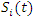’ is given by  as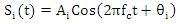(1)
where: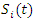is the transmitting signal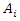is the signal amplitude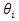is the phase of carrier signal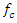is the carrier frequency
t is the symbol period.

### 3. Methodology

The ICI self-cancellation is incorporated in Orthogonal Frequency Division Multiplexing (OFDM). The analysis is carried out over Rayleigh fading channel. Mary-Quadrature Modulation (M-QAM) is used to modulate the transmitted signal. M is the constellation size which is varies in this work to determine the effect of constellation size. Close form expression of the received signal for the proposed technique is derived using Probability Density Function (PDF). The PDF derived is used to obtained the Bit Error Rate (BER) and Spectral Efficiency (SE) to evaluate the performance. Extensive simulations using MATLAB R2018a is used to investigate the performance of the system.

#### 3.1. Incorporation of ICI Self-Cancellation Technique to OFDM System

The high-speed serial signal stream is divided into a set of low speed sub-stream using serial to parallel converter. Inverse Fast Fourier Transform (IFFT) is used to modulate the low speed signal streams onto the orthogonal carrier. The input data symbol is modulated onto a group of subcarriers with predefined coefficients such that the generated ICI signals within that group cancel each other. In this technique, one data symbol is not modulated into one sub-carrier, but at least into two consecutive sub-carriers. If the data symbol ‘r’ is modulated into the first subcarrier, then ‘-r’ is modulated in to the second sub carrier. Therefore, the ICI generated between the two sub- carriers mutually cancels each other at the receiver. The discrete signal is then demodulated using Fast Fourier Transform (FFT) and the signal realised is made to pass through a parallel-to-serial converter as shown in Fig. 1.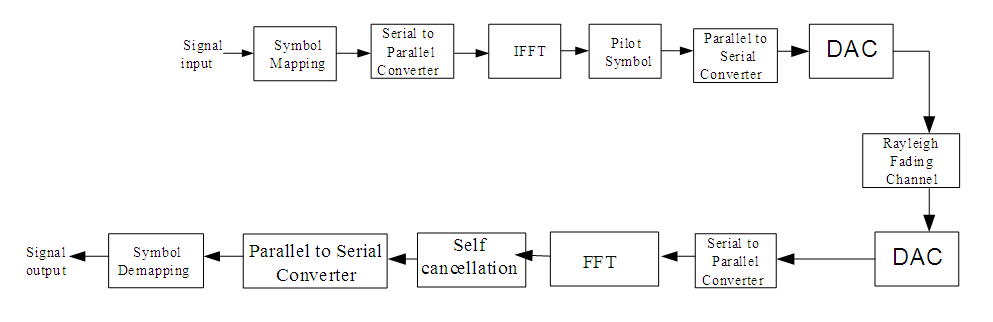Figure 1. Block diagram of OFDM incorporating ICI Self Cancellation Technique
The received signal of N subcarrier OFDM with ICI self-cancellation is given by [21,25] as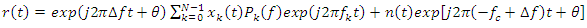(2)
where: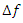is the carrier frequency offset between the transmitter and receiver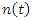is the additive complex Gaussian noise with zero mean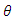is the phase error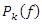is the coefficient of self-cancellation

#### 3.2. Probability Density Function (PDF) of the Received Signal for ICI Self-Cancellation OFDM System

The PDF of the Rayleigh distributions denoted by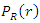is given  as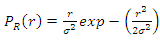(3)
The received signal of the OFDM with ICI self-cancellation is given in equation 1. Therefore, substituting equation (2) into (3) gives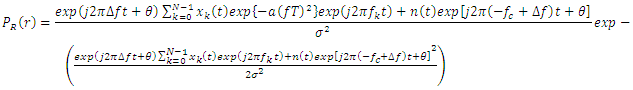(4)
Equation (4) is the PDF of the received signal for the system model.

#### 3.3. Bit Error Rate (BER)

The expression for Bit Error Rate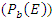is given by  as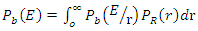(5)
where: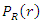is the PDF of the output SNR for the system model.
The conditional error probability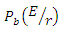is given as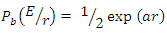(6)
where: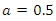for non-coherent modulation
For non-coherent modulation, equation (6) becomes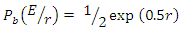(7)
Substituting Equation (7) into (5) gives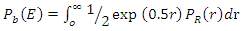(8)
Therefore, substituting equation (4) into (8) gives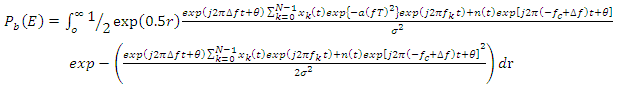(9)

#### 3.4. Spectral Efficiency (SE)

Spectral Efficiency ‘SE’ is given as  as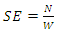(10)
where: N is the capacity of channel
w is the bandwidth of the channel
But according to , capacity of channel ‘w’ is given as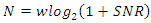(11)
Substituting equation (11) into (10) gives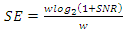(12)
By substituting equation (4) into (12), The SE is obtained as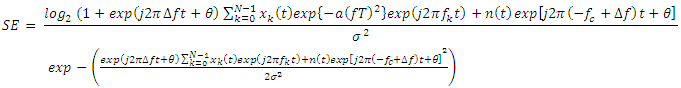(13)
Equation (13) is the Spectral Efficiency (SE) for the system.

### 4. Simulation Results

The evaluation of higher constellation in OFDM with ICI self-cancellation is carried using Bit Error Rate (BER) and Spectral Efficiency (SE). Figs. 2 to 5 present the Bit Error Rate (BER) against SNR for OFDM and OFDM with self-cancellation (OFDM-SC) at different constellation size over Rayleigh fading channel. Fig. 2 depicts BER against SNR for OFDM and OFDM-SC at 16QAM modulation scheme. At SNR of 6 dB, the BER values of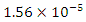and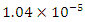are obtained for OFDM and OFDM-SC, respectively, while the corresponding BER values of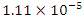and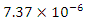are obtained for OFDM and OFDM-SC, respectively, at SNR of 8 dB. Fig. 3 depicts the BER values obtained against SNR for OFDM and OFDM-SC at 32QAM. The BER values of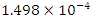and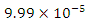are obtained for OFDM and OFDM-SC at SNR of 6 dB. The results obtained revealed that, the BER values for both OFDM and OFDM-SC reduces as SNR increases. The values of BER obtained against SNR for OFDM and OFDM-SC at 64QAM is shown in Fig. 4. At SNR of 8 dB, BER values of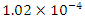and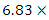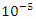are obtained for OFDM and OFDM-SC, respectively. Fig. 5 shows BER values obtained for OFDM and OFDM-SC at 128QAM. The BER values of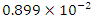and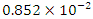for OFDM and OFDM-SC, respectively, at SNR of 8 dB. The results obtained revealed that, OFDM-SC gives lower BER when compared with OFDM and this is due to ICI reduction that reduce error at the receiver. Also, the results obtained revealed that, the BER values obtained increase as constellation size of modulation increases. The percentage increases in BER as between 16QAM and 128QAM is 12%. However, at all the constellation size considered, OFDM-SC shows a better performance with low BER when compared with the conventional OFDM and this is due to the self-cancellation nature of OFDM-SC technique that improves its performance.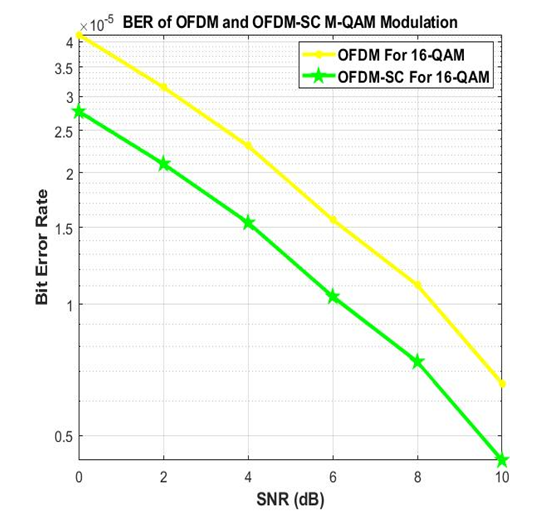Figure 2. Bit Error Rate (BER) for OFDM and OFDM-SC at 16-QAM modulation scheme over Rayleigh fading channel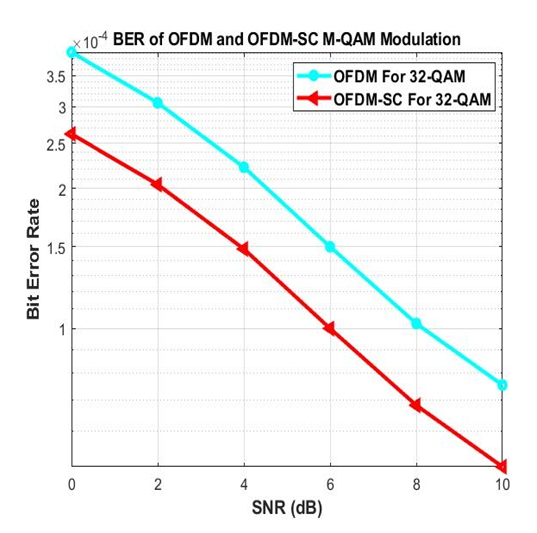Figure 3. Bit Error Rate (BER) for OFDM and OFDM-SC at 32-QAM modulation scheme over Rayleigh fading channel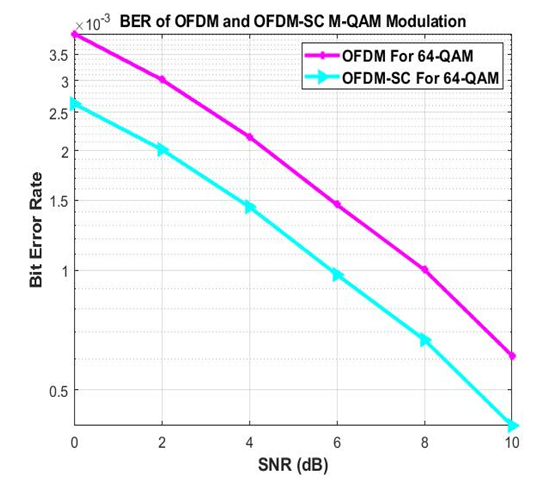Figure 4. Bit Error Rate (BER) for OFDM and OFDM-SC at 64QAM modulation scheme over Rayleigh fading channel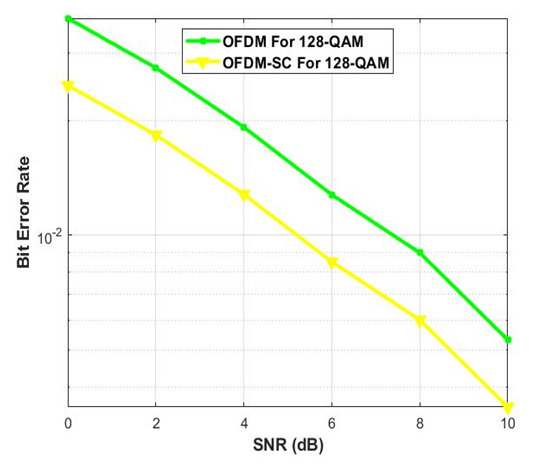Figure 5. Bit Error Rate (BER) for OFDM and OFDM-SC at 128QAM modulation scheme over Rayleigh fading channel
Figs. 6 to 9 depict the Spectral Efficiency (SE) values obtained against SNR for OFDM and OFDM-SC at different constellation size. Fig. 6 shows the SE values obtained against SNR for OFDM and OFDM-SC at 16QAM modulation scheme. The SE values obtained at SNR of 6 dB are 3.8110 and 2.9181 for OFDM and OFDM-SC respectively, while, the corresponding SE values obtained at SNR of 16 dB are 4.7253 and 3.8325, respectively. The SE values obtained for OFDM and OFDM-SC at 32QAM modulation scheme is depicted in Fig. 7. At SNR of 6 dB, SE values of 8.9988 and 8.1060 are obtained for OFDM and OFDM-SC, respectively, as against 11.2848 and 10.3919 obtained at SNR of 16 dB. The results obtained revealed that, SE increases as SNR increases. Fig. 8 depicts the SE values obtained for OFDM and OFDM-SC at 64QAM modulation scheme. At SNR of 6 dB, SE values of 10.7986 and 9.7272 are obtained for OFDM and OFDM-SC, respectively, while the corresponding values obtained at 128QAM modulation scheme are 13.1051 and 11.8048 for OFDM and OFDM-SC, respectively. The results obtained revealed that, at all the constellation considered, OFDM-SC technique shows a reduction in bandwidth efficiency with low SE values due to self-cancellation that consumes additional bandwidth. The results obtained also revealed that, for both OFDM and OFDM-SC, bandwidth efficiency increases as constellation size increases unlike in the case of BER in which error rate decreases as constellation size decreases. The increase in SE values is due to higher constellation that allows transmission of multiple bits at a time. The percentage increase in the value of SE between 16QAM and 128QAM is 68.38%.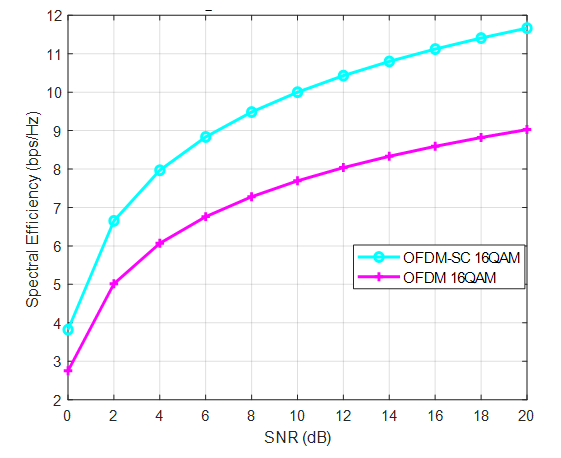Figure 6. Spectral Efficiency (SE) for OFDM and OFDM-SC at 16QAM modulation scheme over Rayleigh fading channel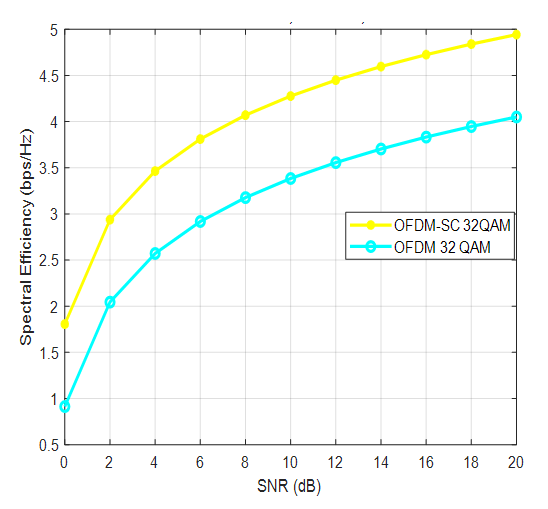Figure 7. Spectral Efficiency (SE) for OFDM and OFDM-SC at 16QAM modulation scheme over Rayleigh fading channel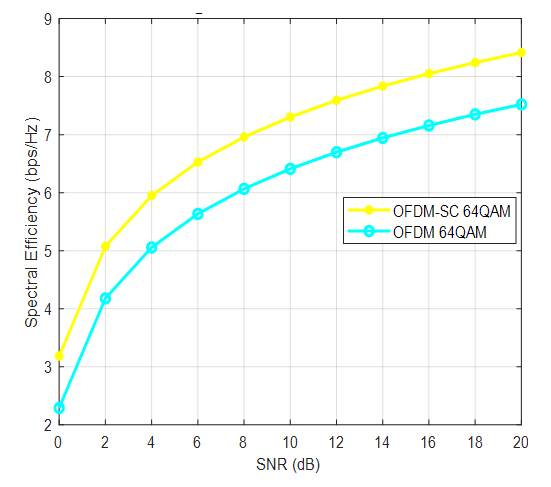Figure 8. Spectral Efficiency (SE) for OFDM and OFDM-SC at 16QAM modulation scheme over Rayleigh fading channel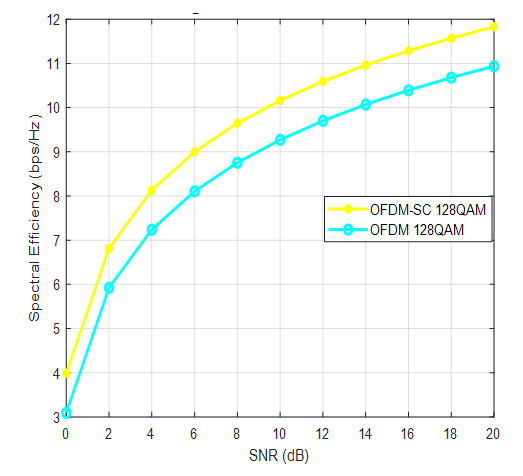Figure 9. Spectral Efficiency (SE) for OFDM and OFDM-SC at 128QAM modulation scheme over Rayleigh fading channel

### 5. Conclusions

Comparative analysis of higher constellation is carried out in this paper. The performance of OFDM and OFDM-SC is carried using Bit Error Rate (BER) and Spectral Efficiency (SE) as performance metrics. Mathematical expression of BER and SE are derived using the signal strength of the receive signal. BER is used to measure the error rate while SE is used to measure the bandwidth efficiency of the two techniques at different constellation size. The results obtained revealed that, OFDM-SC has a better performance in term of error reduction with low BER when compared with conventional OFDM. However, there is reduction in bandwidth efficiency of OFDM-SC with low SE values when compared with conventional OFDM. The results obtained also revealed that, at the two techniques, BER values increases as constellation size increases, while, bandwidth efficiency increase with increases in constellation size. The percentage increase in BER between 16QAM and 128QAM is 12%, while the percentage increase in bandwidth efficiency is 68.38%. Therefore, OFDM-SC with higher constellation is recommended to be used since the percentage increase in bandwidth efficiency is far higher than percentage increase in error rate when using higher constellation. Therefore, OFDM-SC with higher constellation has been shown to have better performance with high bandwidth efficiency. It is concluded that, whenever OFDM-SC is to be used in wireless system, a higher constellation size of modulation such 128QAM and 64QAM must be used to modulate the base band signal.